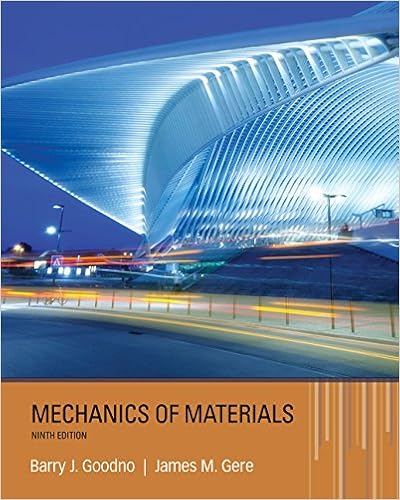# When x 1 this series becomes 1 1 n n n which is

• Test Prep
• 7
• 100% (3) 3 out of 3 people found this document helpful

This preview shows page 3 - 6 out of 7 pages.

##### We have textbook solutions for you!
The document you are viewing contains questions related to this textbook.The document you are viewing contains questions related to this textbook.
Chapter 8 / Exercise 8.2-3
Mechanics of Materials
Gere/GoodnoExpert Verified
1.
##### We have textbook solutions for you!
The document you are viewing contains questions related to this textbook.The document you are viewing contains questions related to this textbook.
Chapter 8 / Exercise 8.2-3
Mechanics of Materials
Gere/GoodnoExpert Verified
MAT1322-3X Solution to Final Examination Summer 2017 4 8. Let z= xe2x−y. Then, when x= 1 and  = y= 2,
(F) −6.
zxy
Part II. Long Answer Questions (26 marks) 1. (4 marks)Find the centroid of the region between the graph of y= 21xand the x-axis, 1 2. x
2
2
2. (4 marks) Suppose a triangular surface is vertically submerged into water (density = 1000 kg / m3) so that the top is 2 meters under the water surface as shown in the following figure: water level2 m8 m5 m
MAT1322-3X Solution to Final Examination Summer 2017 5 Find the total force, in Newtons, acting on this surface. (Use g= 9.81 m /sec2).
) =
F
7
50
3.(4 marks) Air with 0.05% carbon dioxide flows into a room with volume 200 m3at a rate 2 m3/ min and well mixed air flows out at the same rate. Let Q(t) be the amount of carbon dioxide, in m3, in the room at time t(a) (2 marks) Construct a differential equation that is satisfied by the function Q(t(b) (2 marks) Assume, at time t= 0, the air in the room contains 0.2% carbon dioxide. Solve this initial-value problem to find the function Q(t). . ).
•••